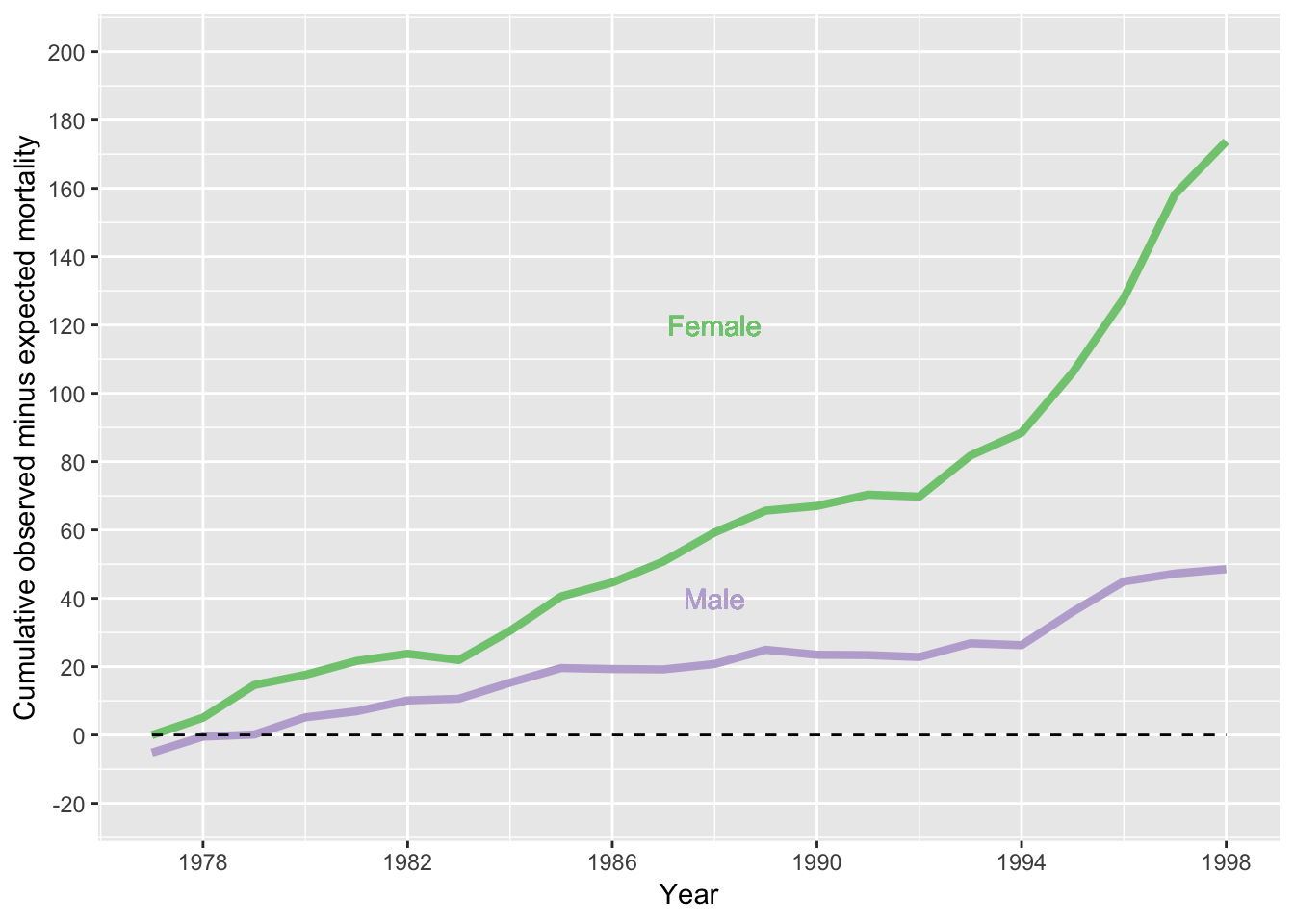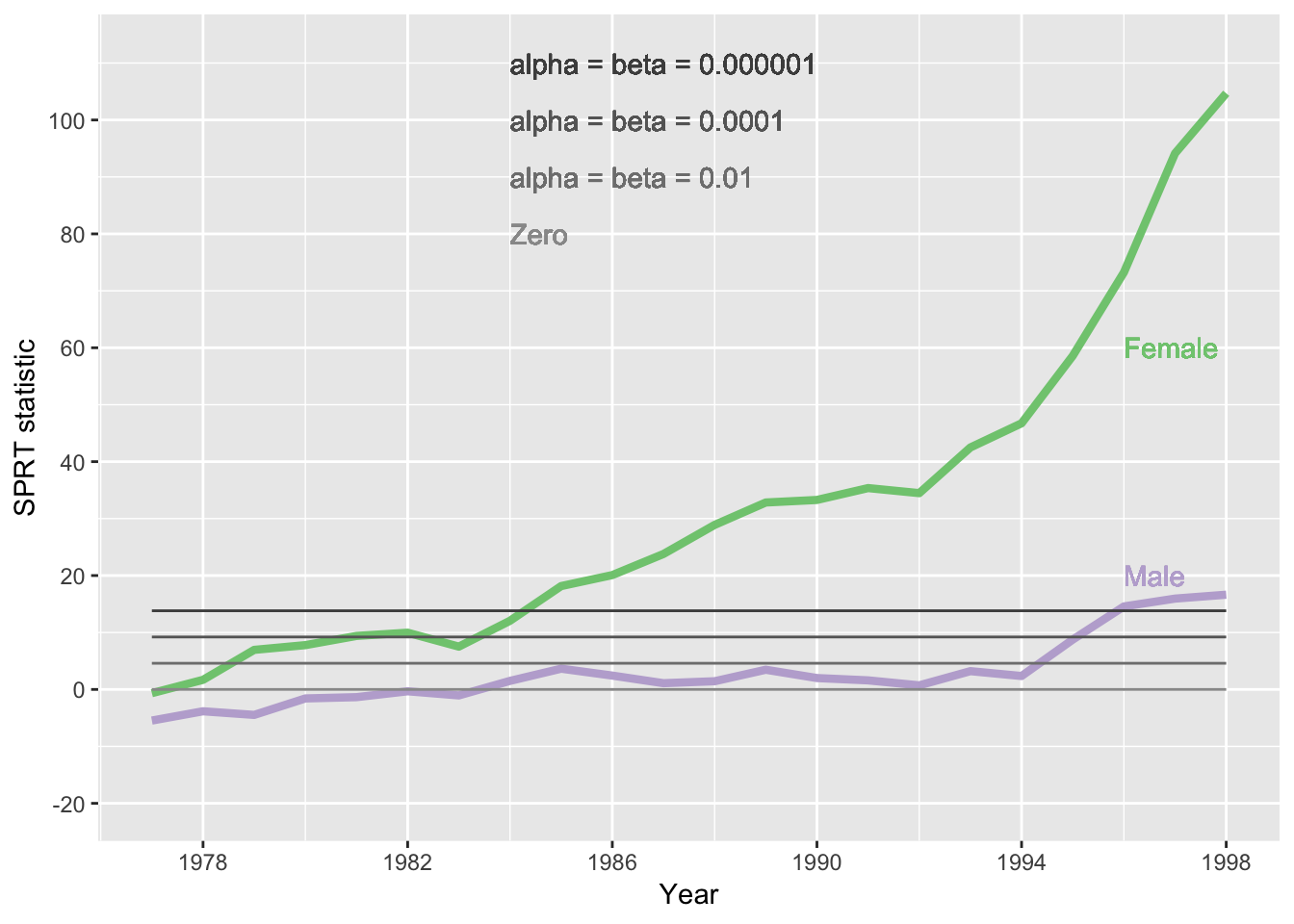Data are for death certficates issued for deaths occurring in the patient’s home or on practice premises, and are contained in 10-3-shipman-cumulative-x.csv, and taken from [Harold Shipman’s Clinical Practice 1974-1998: A Clinical Audit Commissioned by the Chief Medical Officer, by Richard Baker, pages 81-84, Tables 8.23, 8.24, 8.25, 8.26] 5.2](https://webarchive.nationalarchives.gov.uk/20090808160000/http://www.the-shipman-inquiry.org.uk/ge_doclist.asp?ID=5)

### Figure 10.3 Cumulative observed mminus expected deaths

library(ggplot2)

# 10-3
p <- ggplot(df, aes(x=Year)) #+ xlim(1977,1998)
p <- p + geom_line(aes(y=CumWomen65plusObsMinusExpected ,col="Female"), size=1.5)
p <- p + geom_line(aes(y=CumMen65plusObsMinusExpected,col="Male"),size=1.5)

p <- p + expand_limits(y=-20,200)
p <- p + geom_text(aes(x = 1988, y = 120, label = "Female", color = "Female"))
p <- p + geom_text(aes(x = 1988, y = 40, label = "Male", color = "Male"))
p <- p +  labs(x="Year", y="Cumulative observed minus expected mortality")
p <- p + scale_colour_brewer(palette = "Accent") # sets the colour palette
p <- p + scale_x_continuous(breaks=seq(1978, 1998, 4), limits = c(1977,1998))
p <- p + scale_y_continuous(breaks=seq(-20, 200, 20), limits = c(-20,200))
p <- p  + scale_size_continuous(name = "Size", guide = FALSE)  # turns off otherwise added size legend
p <- p + theme(legend.position="none")#, legend.box = "horizontal") # removes the legend
p <- p + geom_line(aes(y=0), linetype="dashed")
pFigure 10.3 number of death certificates signed by Shipman for patients who were age 65 or over and who died at home or in his practice. The expected number, given the composition of his practice list, has been subtracted.

### Figure 10.4 Sequential probability ratio test for Shipman

bookAlpha = bookBeta = c(1/100,1/10000,1/1000000)
#upper boundary for alpha, beta
bookUpper=log((1-bookBeta)/bookAlpha)

p <- ggplot(df, aes(x=Year)) # assign initial plot object, p , using data frame, ship

# add the lines for the SPRT statistic for each gender, and assign them colour names (prepended aa to get the first two assigned palette colours)
p <- p + geom_line(aes(y=log(2)*CumWomen65plusObs-CumWomen65plusExp, colour="aaFemale"), size=1.5)
p <- p + geom_line(aes(y=log(2)*CumMen65plusObs-CumMen65plusExp, colour="aaMale"),size=1.5)
# log(2) is the 0.69 in the book's chapter 10 note 7

# add linerdingly to manipulate the alphabetically assigned colours from the colour palette
p <- p + geom_line(aes(y=0), colour="grey60")#cZero"))
p <- p + geom_line(aes(y=bookUpper), colour="grey50")
p <- p + geom_line(aes(y=bookUpper), colour="grey40")
p <- p + geom_line(aes(y=bookUpper), colour="grey30")
p <- p + expand_limits(y=-20,112) # expanded y-axis limits

# our customised colour legend according to the assigned colours
p <- p + geom_text(aes(x = 1996, y = 60, label = "Female", color = "aaFemale",hjust=0))
p <- p + geom_text(aes(x = 1996, y = 20, label = "Male", color = "aaMale",hjust=0))
p <- p + geom_text(aes(x = 1984, y = 80, label = "Zero", hjust=0), color = "grey60")
p <- p + geom_text(aes(x = 1984, y = 90, label = "alpha = beta = 0.01", hjust=0), color = "grey50")
p <- p + geom_text(aes(x = 1984, y = 100, label = "alpha = beta = 0.0001", hjust=0), color ="grey40")
p <- p + geom_text(aes(x = 1984, y = 110, label = "alpha = beta = 0.000001", hjust=0), color = "grey30")

p <- p +  labs(x="Year", y="SPRT statistic") # Adds title,

p <- p + scale_colour_brewer(palette = "Accent") # sets the colour palette

# define axis limits and label breaks
p <- p + scale_x_continuous(breaks=seq(1978, 1998, 4), limits = c(1977,1998))
p <- p + scale_y_continuous(breaks=seq(-20, 100, 20), limits = c(-20,112))

p <- p  + scale_size_continuous(name = "Size", guide = FALSE)  # turns off otherwise added size legend
p <- p + theme(legend.position="none")#, legend.box = "horizontal") # removes the legend
pFigure 10.4 Sequential Probability Ratio Test (SPRT) statistic for detection of a doubling in mortality risk: patients aged >64 and dying in home/ practice. The straight lines indicate thresholds for ‘alerting’, which provide the overall Type 1 (alpha) and Type II (beta) error rates that are shown - these are assumed to be equal. Looking at the line for females, it is apparent that Shipman would have crossed the outer threshold in 1985.